SSC CGL Previous Year Questions: Number System and HCF & LCM- 2

# SSC CGL Previous Year Questions: Number System and HCF & LCM- 2 - SSC CGL

Test Description

## 30 Questions MCQ Test SSC CGL (Tier - 1) - Previous Year Papers (Topic Wise) - SSC CGL Previous Year Questions: Number System and HCF & LCM- 2

SSC CGL Previous Year Questions: Number System and HCF & LCM- 2 for SSC CGL 2023 is part of SSC CGL (Tier - 1) - Previous Year Papers (Topic Wise) preparation. The SSC CGL Previous Year Questions: Number System and HCF & LCM- 2 questions and answers have been prepared according to the SSC CGL exam syllabus.The SSC CGL Previous Year Questions: Number System and HCF & LCM- 2 MCQs are made for SSC CGL 2023 Exam. Find important definitions, questions, notes, meanings, examples, exercises, MCQs and online tests for SSC CGL Previous Year Questions: Number System and HCF & LCM- 2 below.
Solutions of SSC CGL Previous Year Questions: Number System and HCF & LCM- 2 questions in English are available as part of our SSC CGL (Tier - 1) - Previous Year Papers (Topic Wise) for SSC CGL & SSC CGL Previous Year Questions: Number System and HCF & LCM- 2 solutions in Hindi for SSC CGL (Tier - 1) - Previous Year Papers (Topic Wise) course. Download more important topics, notes, lectures and mock test series for SSC CGL Exam by signing up for free. Attempt SSC CGL Previous Year Questions: Number System and HCF & LCM- 2 | 30 questions in 30 minutes | Mock test for SSC CGL preparation | Free important questions MCQ to study SSC CGL (Tier - 1) - Previous Year Papers (Topic Wise) for SSC CGL Exam | Download free PDF with solutions
 1 Crore+ students have signed up on EduRev. Have you?
SSC CGL Previous Year Questions: Number System and HCF & LCM- 2 - Question 1

### If X and Y are the two digits of the number 347XY such that the number is completely divisible by 80, then what is the value of X + Y?       (SSC CGL 2017)

Detailed Solution for SSC CGL Previous Year Questions: Number System and HCF & LCM- 2 - Question 1

347XY as 347X0. Since 8 is a factor of 80.
347X0 is divisible by 8. It means last three digits 7X0 is divisible by 8.
Hence, X is 2 or 6
if X = 6, number is 34760. But this is not divisible by 80.
if X = 2, number is 34720, which is divisible by 80.
Therefore, number is 34720 with X = 2 and Y = 0.
∴ x + y = 2 + 0 = 2.

SSC CGL Previous Year Questions: Number System and HCF & LCM- 2 - Question 2

### What least value must be assigned to '*' so that the numbers 451*603 is exactly divisible by 9?        (SSC CGL 1st Sit. 2016)

Detailed Solution for SSC CGL Previous Year Questions: Number System and HCF & LCM- 2 - Question 2

To divide 451 * 603 by 9
(4 + 5 + 1 + * + 6 + 0 + 3) = (19 + *)
(19 + *) must be multiple of 9
∴ 19 + * = 27
* = 8

SSC CGL Previous Year Questions: Number System and HCF & LCM- 2 - Question 3

### The least number which when divided by 6, 9, 12, 15, 18 leaves the same remainder 2 in each case is:       (SSC CGL 2nd Sit. 2015)

Detailed Solution for SSC CGL Previous Year Questions: Number System and HCF & LCM- 2 - Question 3

LCM of 6, 9, 12, 15 and 18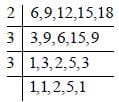LCM = 2 × 3 × 3 × 2 × 5 = 180
Least number = 180 + 2 = 182

SSC CGL Previous Year Questions: Number System and HCF & LCM- 2 - Question 4

The least number that should be added to 2055 so that the sum is exactly divisible by 27 :         (SSC CGL 1st Sit. 2015)

Detailed Solution for SSC CGL Previous Year Questions: Number System and HCF & LCM- 2 - Question 4

Number has to be less than 27. Let the number be x. On Dividing 2055 by 27, we get remainder as 3.
Now, 3 + x = 27
∴ x = 24

SSC CGL Previous Year Questions: Number System and HCF & LCM- 2 - Question 5

If 13 + 23 + ..... + 103 – 3025, then the  value of 23 + 43 +..... + 203 is :       (SSC CHSL 2015)

Detailed Solution for SSC CGL Previous Year Questions: Number System and HCF & LCM- 2 - Question 5

23 + 43 + 63 + ------+ 203
= 23 (13 + 23 + 33 + ------ + 103)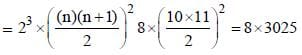= 24200

SSC CGL Previous Year Questions: Number System and HCF & LCM- 2 - Question 6

The smallest five digit number which is divisible by 12, 18 and 21 is :       (SSC CHSL 2015)

Detailed Solution for SSC CGL Previous Year Questions: Number System and HCF & LCM- 2 - Question 6

Lowest 5 digit number = 10,000
The number which is divisible by 12, 18 and 21 is LCM of 12, 18, 12 which is 252.
10000/252 gives 172 as remainder
So, 252 – 172 = 80
10,000 + 80 = 10080
If 10080 when divided by 12, 18 and 21 gives 0 as remainder
So, 10080 is the least 5–digit number.

SSC CGL Previous Year Questions: Number System and HCF & LCM- 2 - Question 7

If the product of first fifty positive consecutive integers be divisible by 7n, where n is an integer, then the largest possible value of n is       (SSC CGL 1st Sit. 2014)

Detailed Solution for SSC CGL Previous Year Questions: Number System and HCF & LCM- 2 - Question 7

Product of first fifty positive consecutive integers = 1 × 2 × .... × 50 = 50 !
Largest possible value of n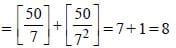SSC CGL Previous Year Questions: Number System and HCF & LCM- 2 - Question 8

The first term of an Arithmetic Progression is 22 and the last term is –11. If the sum is 66, the number of terms in the sequence are :        (SSC CHSL 2014)

Detailed Solution for SSC CGL Previous Year Questions: Number System and HCF & LCM- 2 - Question 8

The sum of Arithmetic Progression is given by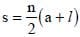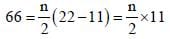n = 12

SSC CGL Previous Year Questions: Number System and HCF & LCM- 2 - Question 9

A teacher wants to arrange his students in an equal number of rows and columns. If there are 1369 students, the number of students in the last row are        (SSC CHSL 2014)

Detailed Solution for SSC CGL Previous Year Questions: Number System and HCF & LCM- 2 - Question 9

If they are equal number of rows and columns then,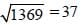SSC CGL Previous Year Questions: Number System and HCF & LCM- 2 - Question 10

The H.C.F. and L.C.M. of  two numbers are 44 and 264 respectively. If the first number is divided by 2, the quotient is 44. The other number is       (SSC CHSL 2014)

Detailed Solution for SSC CGL Previous Year Questions: Number System and HCF & LCM- 2 - Question 10

First number = 2 × 44 = 88
Other number =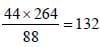SSC CGL Previous Year Questions: Number System and HCF & LCM- 2 - Question 11

Three tankers contain 403 litres, 434 litres, 465 litres of diesel respectively. Then the maximum capacity of a container that can measure the diesel of the three container exact number of times is        (SSC Sub. Ins. 2014)

Detailed Solution for SSC CGL Previous Year Questions: Number System and HCF & LCM- 2 - Question 11

H.C.F. of 403, 434 and 465 is 31.

SSC CGL Previous Year Questions: Number System and HCF & LCM- 2 - Question 12

The next term of the sequence,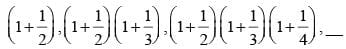is        (SSC Sub. Ins. 2014)

Detailed Solution for SSC CGL Previous Year Questions: Number System and HCF & LCM- 2 - Question 12

Next term will be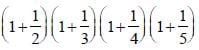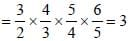SSC CGL Previous Year Questions: Number System and HCF & LCM- 2 - Question 13

Find the number lying between 900 and 1000 which when divided by 38 and 57 leaves in each case a remainder 23.       (SSC Multitasking 2014)

Detailed Solution for SSC CGL Previous Year Questions: Number System and HCF & LCM- 2 - Question 13

L.C.M of (38, 57) = 114
Multiple of 114 between 900 and 1000 = 912
number which leaves 23 = 912 + 23 = 935

SSC CGL Previous Year Questions: Number System and HCF & LCM- 2 - Question 14

The sum of the squares of the  digits of the largest prime number in two digits is          (SSC Multitasking 2014)

Detailed Solution for SSC CGL Previous Year Questions: Number System and HCF & LCM- 2 - Question 14

Largest two digit prime number is 97
92 + 72 = 81 + 49 = 130

SSC CGL Previous Year Questions: Number System and HCF & LCM- 2 - Question 15

A number x when divided by 289 leaves 18 as the remainder. The same number when divided by 17 leaves y as a remainder. The value of y is      (SSC CGL 2nd Sit. 2013)

Detailed Solution for SSC CGL Previous Year Questions: Number System and HCF & LCM- 2 - Question 15

Here, the first divisor (289) is a multiple of second divisor (17).
∴ Required remainder = Remainder obtained on dividing 18 by 17 = 1

SSC CGL Previous Year Questions: Number System and HCF & LCM- 2 - Question 16

Product of two co-prime numbers is 117. Then their L.C.M. is       (SSC CGL 2013)

Detailed Solution for SSC CGL Previous Year Questions: Number System and HCF & LCM- 2 - Question 16

HCF of two-prime numbers = 1
∴ Product of numbers = their LCM = 117

SSC CGL Previous Year Questions: Number System and HCF & LCM- 2 - Question 17

The fifth term of the sequence for which t1 = 1, t2 = 2 and tn + 2 = tn + tn + 1, is      (SSC CGL 1st Sit. 2013)

Detailed Solution for SSC CGL Previous Year Questions: Number System and HCF & LCM- 2 - Question 17

tn + 2 = tn + tn + 1
t2 = t2 + t2 = 3
t4 = t3 + t2 = 3 + 2 = 5
t5 = t4 + t3 = 3 + 5 = 8

SSC CGL Previous Year Questions: Number System and HCF & LCM- 2 - Question 18

If the sum of the digits of any integer lying between 100 and 1000 is subtracted from the number, the result always is       (SSC CHSL 2013)

Detailed Solution for SSC CGL Previous Year Questions: Number System and HCF & LCM- 2 - Question 18

(100x + 10y + z) – (x + y + z) = 99x + 9y
= 9 (11x + y)

SSC CGL Previous Year Questions: Number System and HCF & LCM- 2 - Question 19

'a' divides 228 leaving a remainder 18. The biggest two-digit value of ‘a’ is       (SSC CHSL 2013)

Detailed Solution for SSC CGL Previous Year Questions: Number System and HCF & LCM- 2 - Question 19

228 – 18 = 210 is exactly divisible biggest two digit no. i.e. 70

SSC CGL Previous Year Questions: Number System and HCF & LCM- 2 - Question 20

L.C.M. of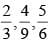is        (SSC CHSL 2013)

Detailed Solution for SSC CGL Previous Year Questions: Number System and HCF & LCM- 2 - Question 20

LCM of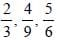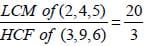SSC CGL Previous Year Questions: Number System and HCF & LCM- 2 - Question 21

The ratio of two numbers is 3 : 4 and their HCF is 5. Their LCM is:        (SSC Sub. Ins. 2013)

Detailed Solution for SSC CGL Previous Year Questions: Number System and HCF & LCM- 2 - Question 21

If the numbers be 3x and 4x, then
HCF = x = 5
∴ Number = 15 and 20
∴ LCM = 12x = 12 × 5 = 60
"Alternate Method:
If two numbers are in the form of 3x and 4x then their L.C.M = 3 ×4 × x
​​​​​​​= 3 × 4 × 5 = 60"

SSC CGL Previous Year Questions: Number System and HCF & LCM- 2 - Question 22

If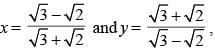then the value of  x3 + y3 is:        (SSC Sub. Ins. 2013)

Detailed Solution for SSC CGL Previous Year Questions: Number System and HCF & LCM- 2 - Question 22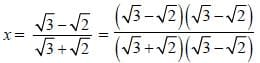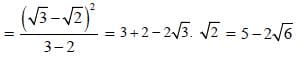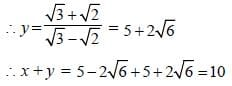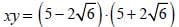= 25 – 24 = 1
∴ x3 + y3 = (x + y)3 – 3xy (x + y)
= (10)3 – 3(10) = 1000–30 = 970

SSC CGL Previous Year Questions: Number System and HCF & LCM- 2 - Question 23

With a two digit prime number, if 18 is added, we get another prime number with digits reversed. How many such numbers are possible?        (SSC CGL 2nd Sit. 2012)

Detailed Solution for SSC CGL Previous Year Questions: Number System and HCF & LCM- 2 - Question 23

Let the number be 10x + y.
According to condition
10x + y + 18 = 10y + x
y – x = 2
So those numbers are 02, 13, 24, 35, 46, 57, 68, 79, 80
But 13 and 79 are prime numbers.

SSC CGL Previous Year Questions: Number System and HCF & LCM- 2 - Question 24

If 13 + 23 + ....... + 93 = 2025, then the approx. value of (0.11)3 + (0.22)3 + ....... + (0.99)3 is         (SSC CGL 2nd Sit. 2012)

Detailed Solution for SSC CGL Previous Year Questions: Number System and HCF & LCM- 2 - Question 24

(0. 11)3 (13 + 23 +...... +93)
= 0 .001331 × 2025
= 1331/40000 ≈ 2.695

SSC CGL Previous Year Questions: Number System and HCF & LCM- 2 - Question 25

The least number which when divided by 48, 64, 90, 120 will leave the remainders 38, 54, 80, 110 respectively, is         (SSC CGL 2nd Sit. 2012)

Detailed Solution for SSC CGL Previous Year Questions: Number System and HCF & LCM- 2 - Question 25

Here, (48 – 38) = 10, (64 – 54) = 10, (90 – 80) = 10 and (120 – 110) = 10.
∴ Required number = (L.C.M of 48, 64, 90 and 120) – 10
= 2870

SSC CGL Previous Year Questions: Number System and HCF & LCM- 2 - Question 26

What would be the sum of 1 + 3 + 5 + 7 + 9 + 11 + 13 + 15 + ....... up to 15th term?       (SSC CGL 2nd Sit. 2012)

Detailed Solution for SSC CGL Previous Year Questions: Number System and HCF & LCM- 2 - Question 26

The sum forms A.P.
First term (a) = 1
Common difference (d) = 2
Sum of 15 term = n/2(2a + (n-1)d)
Sum =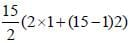= (15/2) x 30 = 225

SSC CGL Previous Year Questions: Number System and HCF & LCM- 2 - Question 27

The least multiple of 13 which when divided by 4, 5, 6, 7 leaves remainder 3 in each case is        (SSC CGL 2nd Sit. 2012)

Detailed Solution for SSC CGL Previous Year Questions: Number System and HCF & LCM- 2 - Question 27

LCM of 4, 5, 6 and 7 = 420
∴ Required number
= 420k + 3 which is exactly divisible by 13.
= 32 × 13k + 4k + 3
Hence, 4k + 3 should be divisible by 13 for some value of k.
For k = 9, 4k + 3 = 39 which is divisible by 13.
∴ Required number = 420 × 9 + 3 = 3783

SSC CGL Previous Year Questions: Number System and HCF & LCM- 2 - Question 28

The next term of the series 1, 5 12, 24, 43 is       (SSC CGL 1st Sit. 2012)

Detailed Solution for SSC CGL Previous Year Questions: Number System and HCF & LCM- 2 - Question 28

The pattern is :
1 + 4 = 5
5 + 7 (= 4 + 3) = 12
12 + 12 (= 7 + 5) = 24
24 + 19 (= 12 + 7) = 43
43 + 28 (= 19 + 9) = 71

SSC CGL Previous Year Questions: Number System and HCF & LCM- 2 - Question 29

The next term of the series – 1, 6, 25, 62, 123, 214, _____ is:        (SSC CGL 1st Sit. 2012)

Detailed Solution for SSC CGL Previous Year Questions: Number System and HCF & LCM- 2 - Question 29

The pattern is :
13 – 2 = –1
23 – 2 = 6
33 – 2 = 25
43 – 2 = 62
53 – 2 = 123
63 – 2 = 214
73 – 2 = 341

SSC CGL Previous Year Questions: Number System and HCF & LCM- 2 - Question 30

The greatest number that will divide 19, 35 and 59 to leave the same remainder in each case is:       (SSC CGL 1st Sit. 2012)

Detailed Solution for SSC CGL Previous Year Questions: Number System and HCF & LCM- 2 - Question 30

Required number = HCF of (35 – 19), (59 – 35) and (59 – 19)
= HCF 16, 24 and 40 = 8

## SSC CGL (Tier - 1) - Previous Year Papers (Topic Wise)

250 tests
Information about SSC CGL Previous Year Questions: Number System and HCF & LCM- 2 Page
In this test you can find the Exam questions for SSC CGL Previous Year Questions: Number System and HCF & LCM- 2 solved & explained in the simplest way possible. Besides giving Questions and answers for SSC CGL Previous Year Questions: Number System and HCF & LCM- 2, EduRev gives you an ample number of Online tests for practice

250 tests